Chirality in Drugs

Many drugs are optically active compounds.  Often it is only one enantiomer that has the desired therapeutic effect.  Thalidomide, a drug used to treat morning sickness in pregnant women in the 1960s is an example of this.The two enantiomers of Thalidomide

The R isomer (pictured on the right above) acts as a sedative while the S isomer (pictured on the left) has teratogenic properties that resulted in thousands of children being born with deformities.

Thalidomide was produced and sold as a racemic mixture of the two enantiomers which resulted in the birth defects.  Even if it was produced in a pure enantiomeric form, this wouldn’t have solved the problem as there is evidence that the enantiomers can convert under physiological conditions.  Mass producing pure enantiomeric forms of drugs can be expensive and low yielding.

Taxol is another drug that has a complex level of chirality.  In total, Taxol has 11 asymmetric carbon atoms.  Taxol is used to treat cancer and is a naturally occurring substance that is found in the bark of Yew trees.The structure of Taxol

Obtaining Taxol from its natural source is extremely low yielding and causes environmental damage as it destroys the trees.  Synthesising the drug from scratch in the laboratory would require a large multistep process that would also prove low yielding and extremely costly.

As such, a compromise is found and the drug is produced by a semi-synthetic route starting with a material found in the needles of the Yew tree.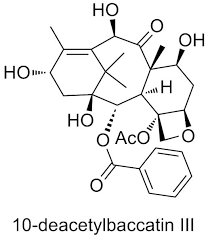This is a more advantageous method as harvesting the needles of the tree does not cause as much environmental damage and significantly lessens the number of steps needed to manufacture the final product.  In addition, the overall yield of Taxol is improved compared to extraction from the bark.

The challenge in the synthesis is creating the correctly oriented chiral centres.  This is done through a method known as asymmetric synthesis.

Asymmetric synthesis involves using a chiral auxiliary attached to the molecule to force through steric hindrance the predominance of one enantiomer over another.

Consider the achiral molecule below.  It is possible to make two enantiomers by adding an amino group to carbon 2.

If you want to favour the production of one enantiomer over the other, attaching a chiral auxiliary molecule to force the addition of the amino group by blocking the path of attack from one side of the molecule.  Below is an example of one such chiral auxiliary.

Notice how the addition of the chiral auxiliary hinders attack from one side of the molecule.  This doesn’t eliminate the production of the unwanted enantiomer but greatly decreases it.

Once the reaction is complete, the chiral auxiliary can be removed for reuse and the final product isolated.

Atomic Structure

History of Atomic Theory

Watch the video below.

✍️    In your notebook, draw an annotated diagram to represent the ideas on the structure of the atom from each of the following scientists:

1. Democritus
2. Dalton
3. Rutherford
4. Bohr
5. Heisenberg

Structure of the Atom

What does an atom mainly consist of?

✍️   To get an idea of the proportions within an atom, look at the following video.  After watching it, write a brief summary of the main points in your notebook.

✍️   Examine table 4 in your data booklet.  How much more mass does a proton or neutron have compared to an electron?

Take a look at the figure below describing nuclear symbols.What is the difference between the mass number and the atomic number?

✍️  For each of the three examples above, write down the number of protons, electrons and neutrons represented by the notation.

Below is a simulation that can be used to practice this notation.  Choose the game option and try all the puzzles.  Don’t move to the next puzzle until you get 5 out of 5!

Electromagnetic SpectrumElectromagnetic Spectrum

Table 3 in your data booklet has a version of the electromagnetic spectrum.

✍️  Using Table 3 and the graphic above, describe the relationships between colour, wavelength, frequency and energy across the spectrum.  Make a summary in your notebook.

Find an example of:

• an emission spectrum
• a line spectrum
• an absorption spectrum

What are the key differences and similarities?
Which of the above were we looking at when we viewed the purple light given off from the hydrogen sample through the diffraction grating in our last class?

Watch the two videos below on the emission spectrum of hydrogen and read the section in your text.  Make a summary for discussion in class tomorrow.

Video #1

Video #2

At the end of this animation, you can try the different transitions yourself.

1.  Oxidation and Reduction

Oxidation and reduction can be considered in terms of:

• oxygen gain or hydrogen loss,
• electron transfer OR
• change in oxidation number

Consider the following reaction:

Zn(s)  +  Cu2+(aq)  –>  Zn2+(aq)  +  Cu(s)

Take a look below for a model of what is happening on an atomic level.

✍️  Looking at the change in charges, which species is gaining electrons and which species is losing electrons?

✍️  Define the terms oxidising agent and reducing agent.  Identify which is which in the above reaction.

Oxidation Numbers

To work out which species is being oxidised and which is being reduced, it is possible to assign oxidation numbers.  Below is a short summary of the rules for assigning oxidation numbers. Alternatively, click here.

✍️  For each of the following, work out the oxidation number of the underlined element

1. N2O4
2. N2
3. NO3
4. NH4+
5. NO2

You can use oxidation number to decide which species is oxidised and which species is reduced in a reaction.

Oxidation number increases = species is oxidised
Oxidation number decreases = species is reduced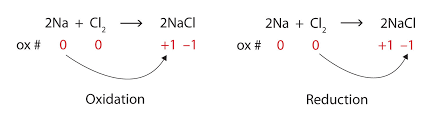✍️  Try question numbers 1-14 on the worksheet found here.  Answers are at the bottom of the link.

One of the properties of transition metals, is that they can have variable oxidation states.  For example find the oxidation numbers for the metal in the following pairs of compounds.

1. CuCl and CuCl2
2. Fe2O3 and FeO

To name these compounds using the IUPAC system we must use the oxidation number of the metal as a Roman numeral.  Eg:

CuCl = copper(I) chloride
CuCl2 = copper(II) chloride

✍️  Write the IUPAC names of the two iron oxide compounds above.

Reactivity of Metal

Table 25 of the Data Booklet ranks the metals in order of increasing activity.  This ranking has the most reactive metals at the top and the least at the bottom.

A metal higher on this list will displace a metal lower on the list from solution.

For example, Zn is above Cu.  As we saw in class, the zinc metal displaced the copper metal from solution.  This is because zinc is more reactive than copper and therefore more easily oxidised.

✍️  Write an equation for the reaction (if one occurs) between the following pairs of metals and ions:

1. magnesium metal and copper sulfate solution
2. silver metal and zinc sulfate solution
3. lead metal and copper sulfate solution
4. tin metal and chromium sulfate solution
5. aluminium metal and nickel sulfate solution

Oxidation and Reduction Half Equations

All redox reactions can be split into their component half-reactions.  Taking the initial example above with the Zn and Cu2+:

Oxidation 1/2 equation: Zn –> Zn2+ + 2e
Reduction 1/2 equations: Cu2+ + 2e –> Cu

Take a look at the rules for balancing half equations in neutral and acidic conditions.  (You can also balance half equations in basic conditions but this isn’t covered in our course).

✍️   After studying these rules, try questions 15 and 16 from the worksheet found here.

Redox Titrations

In our next lesson, we will carry out a redox titration.  In order to practice the calculation and to understand the process, try a few of the problems found at the link below.  Working is given for you if you get stuck but try to do the problems before looking through the answers!

Redox titration problems.

Winkler Method

As an application of redox, the Winkler Method is used to measure biological oxygen demand (BOD) in order to test water quality.  We will do this experiment in class later next week.

Why do we need to know this?  Eutrophication is a problem that has been caused by the misuse of fertilizers.

✍️  Watch the video below and construct a sequencing brainframe™ to explain the process.

To test how polluted a waterway has become, it is necessary to measure the BOD.  This is done by:

1. Testing the dissolved oxygen concentration in the water sample.
2. Leaving the water sample in the dark for 5 days at a particular temperature.
3. Retesting the dissolved oxygen content.
4. Finding the difference between the two values obtained above.  The bigger the difference, the more polluted the water is.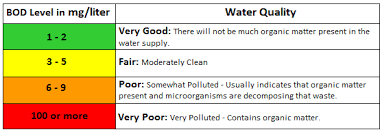Large differences in dissolved oxygen levels can indicate pollution

The Winkler Method can be used to measure the dissolved oxygen concentration.  It used the following three reactions:

1. 2Mn2+(aq) + O2(g) + 4OH(aq) –> 2MnO2(s)  + H2O(l)
2. MnO2(s) +2I(aq) + 4H+(aq) –> Mn2+(aq) + I2(aq) + 2H2O(l)
3. 2S2O32-(aq) + I2(aq) –> 2I(aq) + 2S4O62-(aq)

✍️  What is the mole ratio of O2 : S2O32-?

Watch the slide show to see the different steps and colour changes occurring when you use the Winkler method to determine oxygen concentration.  Try to identify which equation is happening when and what species the colours are due to!

✍️  Read chapter 9.1 of your text book.  Use this and any other sources to add anything important to your notes to make a complete summary of this section.

2.  Electrochemical CellsAn electrochemical cell or voltaic cell can be created to generate electricity.  This is how batteries work.  Above is a simplified diagram of the Daniel Cell which is formed using two half cells – copper and zinc.

✍️   Sketch the diagram in your notebook and then add the following to the diagram:

1. anode
2. cathode
3. + electrode
4. – electrode
5. the 1/2 cell reaction for each cell
6. the direction of electron flow through the wires
7. the direction of ions moving in the salt bridge
8. the overall cell reaction

The above cell can be simplified using cell diagram convention.  It would look like this:

Zn(s)⎮Zn2+(aq) ⎮⎮ Cu2+(aq)⎮Cu(s)

The rules for this type of shorthand is to always put the anode before the cathode and to separate the two solutions by the salt bridge (⎮⎮).

3.  Electrolytic Cells

Electrolysis of a molten salt

Watch the video on the electrolysis of molten lead bromide.  This was the demonstration you saw in class.  Make sure you understand what is happening at the anode and the cathode.

✍️   Predict the products when you electrolyse the following molten salts.  State what you get at the anode and the cathode.
a)  zinc chloride
b)  aluminium oxide

✍️   Draw a diagram for the electrolysis of zinc chloride.  Include the cathode and anode, the direction of electron flow and the half equations that occur at each electrode.  Use the example given in class as a guide.

Electrolysis of Aqueous Solutions (HL)

When you have a molten salt, there is only one possible half reaction able to occur at each electrode.  Once you add water by electrolysing a solution of that salt, you add another species.  Water can be oxidised and/or reduced.  You can find these 1/2 equations in your data booklet.

Possible reactions for a solution of a general salt (MA) being electrolysed:

Cathode  M+ or H2O is reduced               Anode  A or H2O is oxidised

The equation with most positive E is reduced/oxidised in preference.

Electrolysis of Water

Below is the apparatus that can be used to demonstrate the hydrolysis of water.Hoffman Apparatus

1. Dilute acid or sodium hydroxide is used instead of pure water.  Why?
2. The demo is done with dilute sodium hydroxide solution.  Write a list of all the species present in the solution.
3. What are the possible reactions at the cathode?
4. What are the possible reactions at the anode?Watch the video of the electrolysis of water using a dilute sodium hydroxide solution.
1. After observing the demonstration, determine which reaction was taking place at each electrode.
2. Write the overall reaction for the electrolysis of water.

Effects of Concentration and Nature of Electrodes on the Products of Electrolysis

Concentration

Consider the electrolysis of a dilute solution of sodium chloride using graphite electrodes.

✍️  What species are present in solution?

✍️  What possible reactions can occur at the a) anode and b) cathode?  Make a prediction of which reaction you think is most likely to occur.

A dilute solution of sodium chloride with 5 mL of universal indicator was electrolysed for a few seconds.  Below is the before and after pictures of the apparatus.

✍️   From your observations, what products were formed at the anode and cathode?

✍️  What would happen if a concentrated solution of sodium chloride was electrolysed instead?

Nature of the electrode

Consider the electrolysis of a dilute copper sulfate solution with a) inert graphite electrodes and b) copper electrodes.

✍️  For the two situations, predict what the products would be at both the anode and the cathode.  Remember that if you are using a reactive metal like copper as the electrode, then that can also participate in the electrolysis.

Determining the mass of products produced during electrolysis

The amount of products produced depends on the following factors:

• the voltage applied and therefore the current in the circuit
• the time the solution was electrolysed for
• the charge on the ion

A dilute solution of copper sulfate was electrolysed for 20 minutes using a current of 1.50 A.  We can predict the mass of copper metal formed at the cathode.  Consider the following half-equation:

Cu2+(aq) + 2e –> Cu(s)

From this equation, if we know how many moles of electrons we have used in the circuit, then we can find how many moles of copper metal and therefore what mass of metal was deposited.

Step one, is to use the following equation to work out how much charge was supplied in the circuit.

Q = It

where:
Q = charge measured in Coulombs (C)
I  =  current measured in Amperes (A) or Coulombs/second (C s-1)
t  = time measured in seconds (s)

If the current supplied was 1.50 A and the time was 20 minutes, then the charge can be calculated thus:

Q  =  It
=  1.50 x 20 x 60
=  1800 C

Step two, is to work out how many moles of electrons have that much charge.  There is a constant in your data booklet called the Faraday’s Constant (F).  It is the amount of charge carried by 1 mole of electrons and the value is 9.65 x 104 C mol-1.

Therefore we can use the following equation:

n(e)  =  Q / F

where:
n(e)  =  number of moles of electrons measured in moles (mol)
Q = charge measured in Coulombs (C)
F  =  Faraday’s constant measured in Coulombs per mole (C mol-1) and found in your data booklet.

Calculating the number of moles of electrons is as follows:

n(e)  =  Q / F
= 1800 / 9.65 x 104
= 1.9 x 10-2 moles

Step three, uses the mole ratio from the half equation above to determine the number of moles of copper deposited.

n(Cu)  =  1/2 x n(e)
=  1/2  x 1.9 x 10-2
=  9.3 x10-3 moles

Step four, converts moles to mass!

m(Cu)  =  n(Cu) x Ar(Cu)
= 9.3 x 10-3 x  63.55
= 0.59 g

Work through the problems found here.  Your answers may be a little different from those supplied as they use a slightly different value for Faraday’s constant and molar volume of an ideal gas.  You should use the values given by the IB.

Benzene

Review

Discuss with your neighbour everything you remember about the structure of benzene.  Use the diagram below to jog your memory.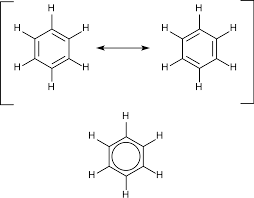Listen to the Voice Thread about Benzene that you created last year.

Review your notes and the section in the text about the structure of benzene before continuing.

Electrophilic Substitution of Benzene

Despite the π-bonds, benzene does not undergo addition reactions like an alkene would.  It does however undergo electrophilic substitution.

✍️  Define the term electrophile and give 3 examples.

Benzene is an electron rich molecule.  This makes it susceptible to attack by electrophiles.  It will react with a mixture of concentrated nitric and sulfuric acids to form nitrobenzene.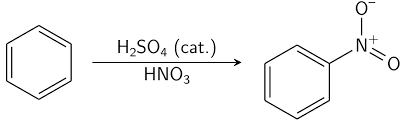Mechanism for the nitration of benzene (HL only)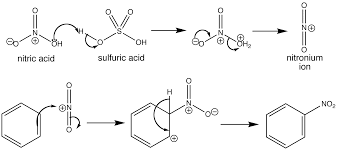✍️  Use your text book (and any other sources you need) to make a complete summary of electrophilic substitution.

Reaction pathways (HL only)

Add the nucleophilic substitution reactions and electrophilic substitution of benzene on to your map.  Remember to add as much detail about conditions as you can.

Halogenoalkanes

Reactivity

Halogenoalkanes are more reactive than alkanes.

Reveiw – Why are alkanes unreactive?  If you can’t answer this question, you need to review alkanes.

✍️  Draw and name all of the isomers of C4H9Br and classify them as primary, secondary and tertiary.

What is different about halogenoalkanes that makes them more reactive than alkanes?  Consider the two points that make alkanes less reactive.  Is there any differences with halogenoalkanes?

Nucleophilic Substitution Reactions of Halogenoalkanes

✍️  Define a nucleophile and give 3 different examples.

We are only going to be concerned with using hydroxide ion (OH) in aqueous solution as our nucleophile.

✍️  (HL) Why is hydroxide ion a better nucleophile than water?  Check and see if you agree with the video below:

When a halogenoalkane is reacted with aqueous OH– an alcohol is produced.✍️  Draw and name the products of nucleophilic substitution with all the isomers of C4H9Br you drew earlier.

We have finished with the standard level material in this section.  Time for review!

Nucleophilic Substitution Mechanisms (HL only)

Depending on whether the halogenoalkane is primary, secondary or tertiary, depends on the mechanism for this reaction.

Primary halogenoalkanes tend to react via a SN2 mechanism.
Tertiary halogenoalkanes tend to react via a SN1 mechanism.
Secondary halogenoalkanes use either and you can’t predict which one.

SN2 MechanismExamine the two mechanisms.  They are written for any halogenoalkane and any nucleophile.

Key to the mechanisms:

L = leaving group.  This is the halogen F, Cl, Br or I.
Nu = nucleophile. This could be OH or any other species with a lone pair.

Now have a look at the following animation.  Here is the link if you want to see the original.
There is more than one type of mechanism here so choose unimolecular nucleophilic substitution for SN1 and bimolecular nucleophilic substituion for SN2.

✍️   After examining the mechanisms and the animation, try and answer the following questions:

• What does S and N stand for in the notation of the mechanism (SN2)?
• The numbers 1 and 2 stand for the molecularity of the mechanism.  What does this mean?
• Define the terms unimolecular and bimolecular.
• Which mechanism has a carbocation intermediate?  Identify it.
• Which mechanism forms a transition state?
• What are the coloured arrows trying to indicate in the SNmechanism?

Conditions for the Reactions

SNreactions are best conducted using protic, polar solvents.
SNreactions are best conducted using aprotic, polar solvents.

Polar, aprotic solvents include:

• propanone
• N,N-dimethylmethanamide
• ethanenitrile

Polar, protic solvents include:

• water
• ammonia
• 2-methylpropan-2-ol
• propan-1-ol and propan-2-ol
• ethanol
• methanol
• ethanoic acid

✍️  Draw the structures for the two groups of solvents.
✍️  What is the difference between an aprotic and a protic solvent?

Rate of Reaction

Examine the two mechanisms again.

✍️  Write a rate equation for each mechanism.

How does the type of halogen affect the rate of reaction?

✍️  Using your data booklet fill in the electronegativities and bond enthalpies for the difference carbon-halogen bonds.

C-X             Electronegativity              Bond enthalpy

C-F
C-Cl
C-Br
C-I

✍️  What trends to you notice?  Discuss these with your table.
✍️  Which halogenoalkane would a nucleophile be most attracted to?

Despite the polarity of the bonds, the most important factor in determining rate is bond strength.

✍️  Knowing this, rank the halogenoalkaness in order from fastest to slowest for reaction with a nucleophile.

Everything you need to know about these two mechanisms is summarised on this sheet here.
✍️   Before trying the review questions, read the relevant section in your text and annotate your notes with any extra important information.

Reduction Reactions (HL)

Just as you can oxidise alcohols to form compounds with a carbonyl group, you can then reduce carbonyl containing compounds back to alcohols.

Reagents for reduction

• Lithium aluminium hydride                 LiAlH4
• Sodium borohydride                             NaBH4Lithium aluminium hydrideSodium borohydride

Structures of the reducing agents

✍️  What difference is there in the conditions under which the two reagents might be used?
✍️   Which reagent is preferred from the reduction of carboxylic acids and why?

Reduction of Carbonyl Containing Compounds✍️  For the following starting materials, draw the structures and name to products formed when treated with lithium aluminium hydride.

• propanoic acid
• butanone

Conversion of nitrobenzene to phenylamine

This reduction happens via a two step process as summarised below.✍️   After reading your text or other sources, elaborate on what is happening in both stages in your notes.  Make sure you can answer these questions.

• Why is a protonated phenylammonium ion produced in the first step?
• What technique is used to heat the reaction?
• What is the role of the tin?
• What is the purpose of using sodium hydroxide in the second stage?

Alcohols

Alcohols as fuels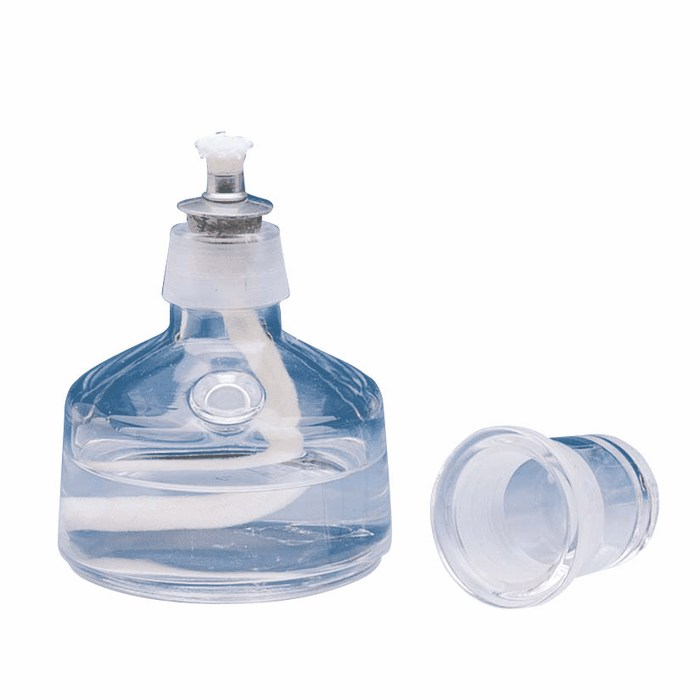The use of ethanol as a fuel is growing around the world. It is hailed as a more environmentally friendly fuel than fossil fuel because the carbon dioxide released from burning the fuel was what the crop absorbed whilst it was growing meaning that no new carbon dioxide has been added to the atmosphere.

Can you see a problem with this logic?  Take a look at the cycle of ethanol production and use below.  How ‘green’ is ethanol as a fuel?Production and Use of Ethanol as a fuel

There has been a lot written about ethanol as an alternative fuel.  If you’re interested, here are a couple of articles with more information:

The complete combustion of ethanol is as follows:

C2H6O(g) + 3O2(g) –> 2CO2(g) + 3H2O(g)

✍️   Write equations for the complete combustion of methanol, propanol and butanol.

Oxidation of Alcohols

1.  Primary, Secondary and Tertiary Alcohols

✍️   Draw the structures and name all the alcohols with molecular formula C4H10O.
✍️   Classify these into primary, secondary and tertiary alcohols.

2.  Common Oxidising Agents

In the next unit (Topic 9/19 of your syllabus) we will discuss these in more detail.  However, for now, we will look at two reagents that are used for oxidising alcohols:

• acidified potassium permanganate (VII)       KMnO4
OR
• acidified sodium dichromate (VI)                   Na2Cr2O7

Either of these two reagents can be used.  It is important to learn what their colours before and after reaction.3.  An Experiment

A student decided to look at what types of alcohols were able to be oxidised.  She decided to use the following alcohols:

• ethanol
• propan-1-ol
• propan-2-ol
• 2-methylpropan-2-ol

✍️  Draw the full structural formula for each of the alcohols above.
✍️  Classify them as either primary, secondary or tertiary.

She decided to try reacting the alcohols with acidified sodium dichromate(VI) in one trial and acidified potassium permanganate(VII) in the other.  She set up the two trials as shown below with these reagents.Acidified potassium permanganate(VII) BEFORE reaction with alcohols.

Into the wells, she put two drops of the following alcohols:

A1 Ethanol
A2 Propan-1-ol
A3 Propan-2-ol
A4 2-methylpropan-2-ol
and
B1 or B2 – no alcohol as this was the control

After 15 minutes, she observed the following changes.

✍️  From her results, which types of alcohols (primary, secondary and/or tertiary) undergo oxidation?
✍️  The tray with the potassium permanganate(VII) showed a reaction but a brown precipitate formed in the wells.  What is this?

For now we aren’t going to worry about trying to balance these redox equations but instead just focus on what happens to the alcohol.

This will depend on whether the alcohol is primary secondary or tertiary.  Below is a diagram representing the different possibilities for the oxidation of alcohols.✍️  After examining the chart, what were the products of the reactions in each of the wells A1, A2, A3 and A4?

4.  Techniques for Oxidising AlcoholsHeating under refluxDistillation

Techniques for heating and recovering products in organic chemistry.

✍️  Using the above chart, what would be the products when the following are oxidised under the conditions specified:

1. Butan-1-ol is reacted with stoichiometrically equivalent amounts of acidified potassium permanganate (VII) and the product is removed by distillation as it is formed.
2. Methanol is reacted with excess acidified sodium dichromate (VI) and heated under reflux before the product is removed by distillation.
3. Butan-2-ol is reacted with excess acidified potassium dichromate (VI) and heated under reflux before the product is removed by distillation.
4. Methylpropan-2-ol is heated under reflux with excess potassium permanganate (VII).

Esterification

Esterification is a type of condensation reaction where an alcohol and a carboxylic acid are combined to form an ester.Some important points to note are:

• this is a reversible reaction so a 100% yield is impossible to obtain
• reaction requires heat
• reaction requires an acid catalyst usually in the form of concentrated sulfuric acid
• esters are often fragrant and many have fruity smells✍️   Write the equation (using structural formula for all organic compounds) between ethanol and butanoic acid.  Name the ester produced.

Reaction Pathways (HL only)

So far we have talked about alkanes, alkenes and alcohols.  We have also made halogenalkanes, aldehydes, ketones, carboxylic acids and esters in our discussions.

✍️  Discuss at your table how you could make ethanoic acid from ethene.  What reagents would you need and under what conditions (heat, reflux, distillation) would you use at each step?

✍️  Construct a map that connects the types of compounds we have discussed so far.  Over the arrows, put the conditions and reagents needed for the reactions.

Alkenes

Reactivity

Alkenes are more reactive than alkanes.  Electrons in π bonds are not as strongly attracted to the nuclei as the electrons in the σ bond.  This makes the π bond weaker.

✍️    HL – What is the hybridisation of carbon in this molecule?  If you can’t answer that question, you need to revise this section from bonding.A model of ethene showing the electron distribution

Distinguishing between alkanes and alkenes

✍️   Review this summary of alkenes and add your own summary to your notes.  Include an example of the reaction that occurs when adding bromine water to an alkene.

✍️  What type of reaction is occurring in the alkene test tube?

✍️   If left overnight, the test tube containing the alkane would also decolourise.  Write a set of equations to explain this and identify the type of reaction occurring.

Alkenes can react with:

• halogens eg F2, Cl2, Br2 and I2
• hydrogen halides eg HF, HCl, HBr and HI and
• water H2O in the presences of a sulfuric acid catalyst
• hydrogen in the presences of a Ni catalyst

✍️  Write the equation for the reaction of but-2-ene with each of the following reactants above.  Use structural formulae in the equations and name the product each time.

✍️  What classes of compounds can be made from addition reactions of alkenes?

Review pages 3 and 4 of this summary and write your own notes.  Make sure you view both the animations.

Key terms to remember here are monomer and polymer.  Make sure you can define both and know how one relates to the other!

Try the polymer puzzles found here.

This concludes the material for standard level.  You should now read the section in your text book (10.2) which is relevant to alkenes and addition polymerisation and add any thing else you find important to your notes.

Electrophilic Addition Reactions – HL only

Study the following image of a general mechanism for electrophilic addition reactions.  What do you think it is showing you?  Think about the following:

• What do you think the curly arrows are representing?
• What does r.d.s stand for?
• Why is the second step faster than the first step?The above diagram is the general mechanism for electrophilic addition of any halogen (X2), halogen halide (HX) or interhalogen (eg I-Cl or iodine monochloride) with any alkene.

✍️  Draw the mechanism for the bromination of ethene.

Remembering that an electrophile is an electron deficient species, how is Br2 considered an electrophile in this mechanism?

Below is the reaction between propene and HBr.As you can see, there are two possible products.  One is more likely than the other.

✍️  Draw the mechanism to create both the products.

The Markovnikov rule explains why 2-bromopropane is the major product.  Simply put the Markovnikov rule is…

“The hydrogen rich get richer!”

✍️  Use your textbook to read about this rule and write a summary to explain why the major product is 2-bromopropane and not 1-bromopropane in terms of the stability of the carbocation.

Post Expedition Reflection

Write a blog post about your experience on expedition.As a guide, reflect on the following questions:

• What did I enjoy?
• What did I do well?
• What challenges did I face?
• What did I learn about living and working in a community?
• How did I grow?
• Why are the Expeditions an important part of our schooling?
• What skill/idea/goal that I began to work on during the expedition, do I want to continue with at school this year?

You might like to include some of the photos taken by the teachers to illustrate your post (try and include relevant images) or include some of your own.